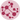# Network Layout Possibilities

Charts in post #320 and #321 explain how to draw a basic network chart and customize its appearance. The next step is to control the layout of your network. This post describes how to do it and provides several examples which different layout algorithms applied.

There is actually an algorithm that calculates the most optimal position of each node. Several algorithms have been developed and are proposed by NetworkX. This page illustrates this concept by taking the same small dataset and applying different layout algorithms on it. If you have no idea which one is the best for you, just let the function to use the default! (It will be the fruchterman Reingold solution). Read more about it with `help(nx.layout)`.

``````# libraries
import pandas as pd
import numpy as np
import networkx as nx
import matplotlib.pyplot as plt

# Build a dataframe with your connections
df = pd.DataFrame({ 'from':['A', 'B', 'C','A','E','F','E','G','G','D','F'], 'to':['D', 'A', 'E','C','A','F','G','D','B','G','C']})
df

G=nx.from_pandas_edgelist(df, 'from', 'to')

# Fruchterman Reingold
nx.draw(G, with_labels=True, node_size=1500, node_color="skyblue", pos=nx.fruchterman_reingold_layout(G))
plt.title("fruchterman_reingold")
plt.show()

# Circular
nx.draw(G, with_labels=True, node_size=1500, node_color="skyblue", pos=nx.circular_layout(G))
plt.title("circular")
plt.show()

# Random
nx.draw(G, with_labels=True, node_size=1500, node_color="skyblue", pos=nx.random_layout(G))
plt.title("random")
plt.show()

# Spectral
nx.draw(G, with_labels=True, node_size=1500, node_color="skyblue", pos=nx.spectral_layout(G))
plt.title("spectral")
plt.show()

# Spring
nx.draw(G, with_labels=True, node_size=1500, node_color="skyblue", pos=nx.spring_layout(G))
plt.title("spring")
plt.show()``````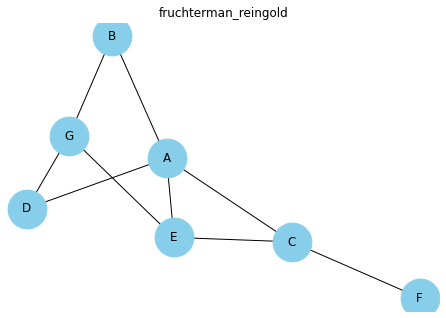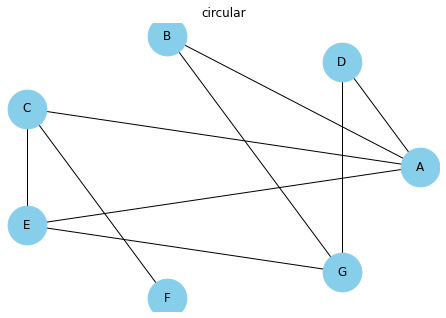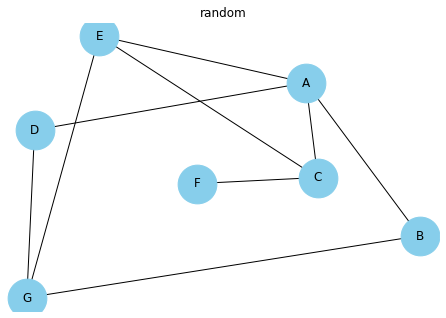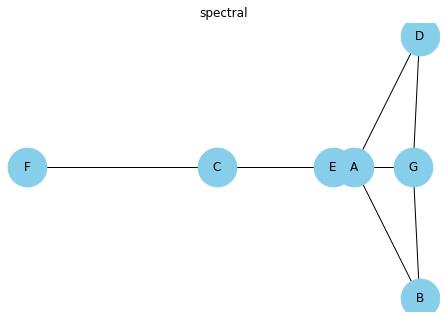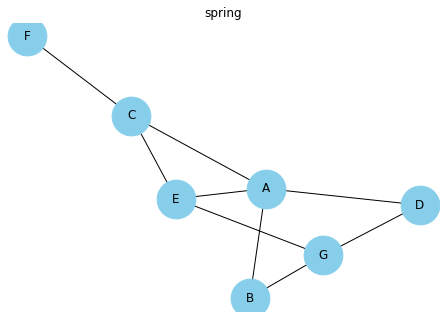## Contact & Edit

👋 This document is a work by Yan Holtz. Any feedback is highly encouraged. You can fill an issue on Github, drop me a message onTwitter, or send an email pasting `yan.holtz.data` with `gmail.com`.